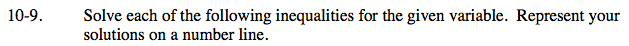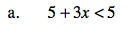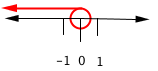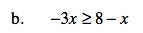### Home > AC > Chapter 10 > Lesson 10.1.1 > Problem10-9

10-9.Find the boundary point. 5 + 3x = 5

Solve for x.
3x = 0
x = 0

Test numbers on both sides of zero.
5 + 3(−1) < 5 5 + 3(1) < 5
5 − 3 < 5 5 + 3 < 5
2 < 5 8 < 5
True FalseFollow the steps in part (a).